Journal of Electrical and Electronic Engineering
Volume 4, Issue 5, October 2016, Pages: 131-138

Closed-Loop Design for Standalone Photovoltaic-Battery Hybrid Power System

Xiong Xiaoling

State Key Laboratory of Alternate Electrical Power System with Renewable Energy Sources (North China Electric Power University), Beijing, China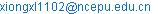Xiong Xiaoling. Closed-Loop Design for Standalone Photovoltaic-Battery Hybrid Power System. Journal of Electrical and Electronic Engineering. Vol. 4, No. 5, 2016, pp. 131-138. doi: 10.11648/j.jeee.20160405.17

Received: November 1, 2016; Accepted: November 22, 2016; Published: November 24, 2016

Abstract: The photovoltaic-battery hybrid power system is constructed with photovoltaic panels and a battery, which are connected to the load via a boost converter and a bidirectional buck/boost converter, respectively. Depending on the amount of available solar power, the photovoltaic panels may operate in a maximum power point tracking (MPPT) mode or off-MPPT mode to regulate the output voltage, and at the same time, the battery may provide power to the load or store energy from the solar power. The whole system is thus designed to operate with multiple operating modes. Compared to the system of single operating mode, the stability analysis and closed-loop design is much more difficult, as the control loops are usually coupled with each other and the design for stable operation of such system requires consideration of the stability conditions for all possible operating modes. To design the closed-loop, the commonly used small-signal analysis method based on averaged state-space is helpless here, and then the nonlinear analysis method with discrete-time mapping model is performed to evaluate the stability boundaries of the system. The parameters of the closed-loop are chosen in the stable region of the stability boundary diagrams. Moreover, a prototype is built and the experimental results are shown to verify the nonlinear analysis method in the design of closed-loop.

Keywords: Renewable Power Generation System, Photovoltaic Panels, Multiple Operating Modes, Nonlinear Analysis, Closed-Loop, Stability

Contents

1. Introduction

In order to avoid the possibility of energy crisis and solve global environment problems, development renewable power generation systems with solar energy, wind energy and so on has gained more and more attention in recent years . However, as climate, solar radiation, wind speed, etc. vary from time to time, the amount of available energy from renewable energy sources is fluctuating and even intermittent. Thus, a power system that depends entirely upon one kind renewable energy source can be unreliable. In order to provide continuous electrical power to the load, multiple renewable energy sources and energy storages, such as batteries, super capacitors, etc, are usually adopted to construct hybrid renewable power generation systems [2,3]. As the power distribution of the multiple sources is controlled reasonably, the stability and flexibility of the system can be improved.

The renewable power generation systems can be grid-connected systems or standalone system . For localized applications in some remote non-electrified regions, constructing stand-alone power generation systems is often more cost-effective than the conventional deployment of the grid-connected power generation systems. The renewable energy sources and energy storages can be connected to a common dc bus or a common ac bus via a number of power converters. In the field of power supply, transportation, communication and so on, the renewable power generation system based on dc bus is more commonly used [5, 6]. Fig. 1 shows one of standalone hybrid renewable power generation system based on a common dc bus, in which photovoltaic (PV) panels are connected the dc bus via a dc/dc power converter, and the energy storage is connected to the dc bus with a bidirectional dc/dc power converter, respectively.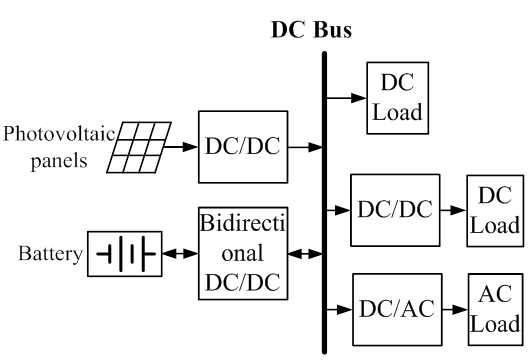Figure 1. Standalone photovoltaic-battery hybrid power system based on dc bus.

There are two sources in Figure 1, and then the power from each source needs to be managed, such as the solar power should be used as much as possible to save up the energy from the back up source, i.e., the battery. At the same time, the energy storage needs to be protected safely from damaging. Then, the PV panels may operate in a maximum power point tracking (MPPT) mode or off-MPPT mode to regulate the output voltage, and at the same time, the battery may provide power to the load or store energy from the PV panels. The whole system is thus designed to operate with multiple operating modes [7-10]. Compared to the system of single operating mode, the stability analysis and closed-loop design is much more difficult, as the control loops are usually coupled with each other and the design for stable operation of such system requires consideration of the stability conditions for all possible operating modes.

To design the parameters of the closed-loop, the commonly used small-signal analysis method based on averaged state-space [11-13] is helpless here. The two reasons are: (1) there are several control loops to regulate the several duty cycles in each operating mode, and the control loops are usually coupled with each other, which make the closed-loop design become difficult. The literature  provided an ideal to design the regulators first in the operating mode without control loop coupling, and then transmitted the designed parameters into other operating modes and checked the stability of the system. If they are not satisfied, the parameters of the regulators need to be adjusted until they meet the stability requirements. However, this method cannot be applied when the control loops are coupled in each operating mode. The literature  introduced a decoupling matrix to design the regulatorsof the coupled control loops, but this method is useless in the multi-operating-mode system, because the decoupling matrixes are different in each operating mode and introduce new couple for another operating mode. (2) The small signal model based on the state space averaging method can capture and analyze the low-frequency oscillation of the system, but cannot predict the instability at and near the switching frequency [15,16]. Therefore, this paper will analyze the stability of the photovoltaic battery hybrid power system (PBHPS) with nonlinear theory base on a discrete-time model. Through this method, the stability boundaries can be found and used to design the parameters of the closed-loop.

In this paper, we will first describe the PBHPS with multiple operating modes and the energy management strategy in Section 2. The nonlinear stability analysis with a discrete-time model is presented in Section 3. The stability boundaries under different choices of compensation parameters are graphically derived in Section 4. The analysis results can thus be used to design the parameters of regulators in the closed loops. In Section 5, we will give the experiment results to verify the nonlinear analysis method. At last, a conclusion is given in Section 6.

2. PBHPS Description

2.1. The Operating Modes of PBHPS

Figure 2 shows the photovoltaic-battery hybrid power system, which provides power to the dc load based on a solar power system and a battery connected to an output dc bus via a boost converter and a bidirectional buck/boost converter, respectively. The solar power is regulated by boost converter, while the bidirectional converter controls the power of the battery. When the solar power is insufficient than the load power, MPPT control strategy for the PVpanels is employed to collect power from the PV panels, and the bidirectional buck/boost converter operates as a boost converter to power the load. When the solar power becomes larger than the load power, the excessive power is used to charge the battery. At this time, if the charge current or charge voltage of the battery achieves the maximum value recommended by the manufacturers, the charge process needs to be controlled with the bidirectional buck/boost converter operating as a buck converter, and meanwhile, the PV panels needs to quit MPPT control and change to regulate the output voltage. Thus, the PBHPS is designed to operate with multiple operating modes.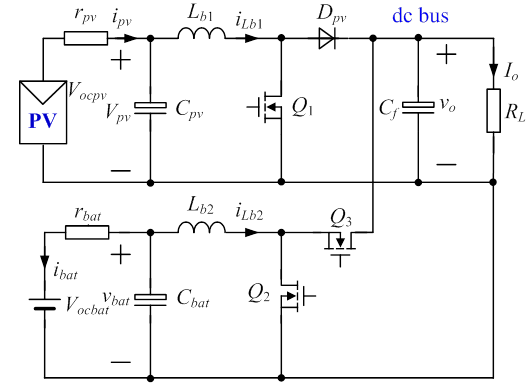Figure 2. Main topology of standalone PBHPS.

According to the maximum power supplied by the PV panels and the state of the battery, the PBHPS has three normal operating modes and one shunt-down mode, as shown in Table 1, where Po is the output power, Pm is the maximum solar power, Vbmax and Ibmax are the recommended maximum charge voltage and charge current, and Vbmin is the permitted minimum battery voltage. vbat and ibat are the voltage and current of the battery, as shown in Figure 2.

Table 1. The operating modes of PBHPS.

 Pm

If Pm<Po and the battery is deeply discharged, the whole system is shut down.

Operating Mode M1 — If Pm<Po and the battery is able to provide power, the PV panels power the load with the MPPT algorithm enabled. In this mode, the input current for the boost converter, i.e. ipv is controlled. The battery provides complementary power through the bidirectional buck/boost converter operating in boost mode to regulate the output voltage vo.

If Pm>Po by a small margin, the excessive solar power is used to charge the battery, and the charge current ibat is smaller than Ibmax. In this case, the control system is the same as operating mode M1. The difference is that the inductor current iLb2 in Figure 2 reverses.

Operating Mode M2 — If Pm>Po and ibat reaches the limit Ibmax, it is necessary to control the charge current to protect the battery, so the bidirectional buck/boost converter operates in buck mode to control the charge current. Meanwhile, the PV panels need to quit MPPT control and regulate the output voltage.

Operating Mode M3 — If Pm>Po and vbat achieves the limit Vbmax, the bidirectional buck/boost converter operates in buck mode to control the charge voltage. Meanwhile, the boost converter still regulates the output voltage.

2.2. The Control System of PBHPS

In this paper, the Perturb & Observe algorithm  is chosen for MPPT implementation, as shown in Figure 3. The output current of the PV panels, denoted as ipv, is compared with a reference current Ipvref which is calculated from the MPPT algorithm. Thus, the loop controls the PV power ppv in the event of any change in duty cycle of boost converter and forces ipv to follow the reference current Ipvref, then the PV panels output the maximum power Pm.

In this paper, the Perturb & Observe algorithm  is chosen for MPPT implementation, as shown in Figure 3. The output current of the PV panels, denoted as ipv, is compared with a reference current Ipvref which is calculated from the MPPT algorithm. Thus, the loop controls the PV power ppv in the event of any change in duty cycle of boost converter and forces ipv to follow the reference current Ipvref, then the PV panels output the maximum power Pm.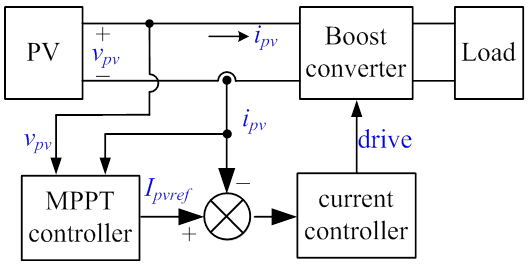Figure 3. MPPT control system.

Figure 4 shows the control system of PBHPS, where Vof = voHof and vbatf= vbatHbf. Here, Hof and Hbf are the sampling coefficients for the output voltage and the battery voltage, respectively. They are proportionality constants implemented by resistor dividers. Also, Ksis the sampling coefficient for inductor current iLb1 and is also a proportionality constant. The compensation ramp Vp shown in Figure 4(b), is applied to increase the stability range of peak current controlled converters. With an additional diode Dc and a resistor R connected to ground, the control loop forthe boost converter can switch from the peak current loop controllingiLb1 to a dual control loop controlling vo. At the same time, Q2 is the mainly controlled switch, regardless of the bidirectional buck/boost converter operating as a boost converter or a buck converter, and the duty cycle of Q3 is always complementary to Q2, so a negative logic is inserted in the charge current andcharge voltage control loops to share the PWM comparator.

When the system operates in M1, diodes Dc, D2 and D3 are off, D1 is on, the input current ipv or iLb1 is controlled with the MPPT algorithm under a peak current control loop, and output voltage vo is regulated through a voltageloop by the bidirectional converter which operates in boostmode. If Pm>Po and ibat reaches the limit Ibmax, then the charge current control loop is enabled, i.e., D2 is on, D1 is off. This will lead to an increase in vo as excessive power is delivered to the load. Thus, the error signal of regulator1, ve1, will drop. When it falls below zero, diode Dc is turned on, and vo is regulated through a dualloopconsisting of an inner peak current loop and an outervoltage loop. In this case, Iref =Ipvref + ve1, then the MPPT algorithm disabled. At this point, the system is switched to M2 smoothly. If Pm>Po and vbat achieves the limit Vbmax, it will enable the charge voltagecontrol loop, causing D3 to turn on and D2 to turn off, and the system switches to M3.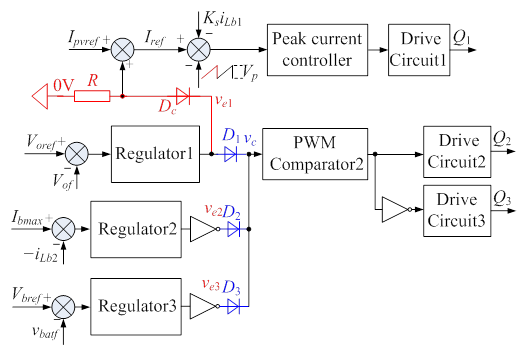(a) The control system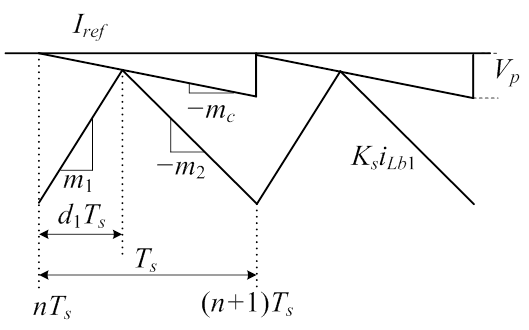(b) Typical waveforms for peak current control loop

Figure 4. The control system of PBHPS.

3. The Discrete-Time Model

As an illustration, the operating mode M1 is analyzed with the discrete-time model in detail, and the same analysis procedure can be applied to other operating modes. In our study, the switching frequency of the two converters is the same and trailing edge modulation is used for "PWM Comparator2". Continuous Current Mode (CCM) operation is assumed, so diode Dpv and switch Q3 are always in complementary states to switches Q1 and Q2. Hence, in one switching period, the system toggles among three switch states and the sequence takes the following order: (i) Q1 and Q2 are on; (ii) Q1 is on and Q2 is off (if d1³d2) or Q1 is off and Q2 is on (if d1£d2); (iii) Q1 and Q2 are off. Here, we assume that d1³d2. Then, the state equations for the main circuit in Figure 2 are(1)

Where q1 and q2 are the switching functions of Q1 and Q2, given as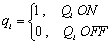(2)

Proportional-Integral (PI) regulators are employed, and the transfer function is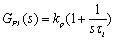(3)

Here kp and τi are the proportional gain and the integral time constant. In addition, kpj and τij (j = 1, 2, 3) are the parameters for "Regulator1", "Regulator2" and "Regulator3" in Figure 4(a), respectively.

In M1, the boost converter operates with MPPT control, Iref=Ipvref, whereas the bidirectional buck/boost converter operates in boost mode to control vo. Thus, according to the control strategy in Figure 4(a), the modulation signal vc is equal to the error signals ve1, and vc satisfy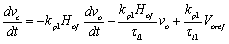(4)

Therefore, the state variable vector of operating mode M1 is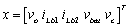. The corresponding state equations for the three switch states are generally given by(5)

Where n= 1, 2, 3 correspond to switch state (i) (ii) and (iii), respectively. E1 is the voltage vector, and is given as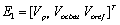, so A’s and B’s are given by(6)(7)

We denote the sampled state value at starting and endinginstants of the nth period as xn= x(nTs), xn+1= x((n+1)Ts), respectively. For each state equation in (5), we can find the solution, i.e.,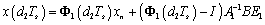(8)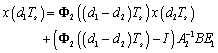(9)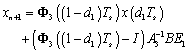(10)

Where I is unit matrix, Fj(x) is the transition matrix and is given by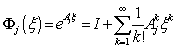(11)

To complete the discrete-time map, the relationship between the duty cycles and the state variables should be derived. During the period of Q1 is on, inductor current iLb1 rises, and when it reaches the reference level Iref, switch Q1 is turnedoff. Thus, the switching function for Q1 can be derived as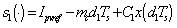(12)

Where C1 = [0 −Ks 0 0 0]and mc is the compensation slope given by mc = Vp/Ts, as shown in Figure 4(b).

In the output voltage control loop, the "PWM comparator2" compares vc with the ramp signal:(13)

Where VL and mramp are the lower threshold and rising slope of the ramp signal, respectively. Basically, Q2 is turned on if vc>vramp, and is off otherwise. Then, we can also get a switching function for Q2, as(14)

Where C2 = ].

Combining (10), (12) and (14), an exact discrete-time model is derived. Suppose the equilibrium point is given by xn = xe. By setting xn+1 = xn = xe, s1 = 0 and s2 = 0, the equilibrium point xe and steady-state duty cycles D1 and D2 can be obtained. Then, the Jacobian of the discrete-time model evaluated at the equilibrium point is(15)

All the eigenvalues of the Jacobian can be obtained with the characteristic equation, as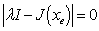(16)

If all the eigenvalues are inside the unit circle, the equilibrium state is stable. Any eigenvalue crossing the unit circle to outside indicate the system becomes unstable.

4. The Stability Boundaries of PBHPS

In our study, the system specifications and the parameters of the converters are chosen, as shown in Table 2.

Table 2. The parameters of PBHPS.

 parameters values parameters values Vpv 20~34/V Ipvref 0~3/A Vocbat 34~40/V rbat 8/mΩ Vbmax 40/V Ibmax 3/A Vo 48/V Io 0~5/A Lb1 48/μH Lb2 48/μH Cf 1.88/mF Cbat 400/μF Ks 0.1 fs 100/kHz

In this section, we will generate stability boundaries information. In general, the PI control parameters and slope compensation Vp are important deciding parameters, as kp need to be set sufficiently largeto reduce the steady-state error while τi should be small toimprove the dynamic response. However, if kp is too large or τi is too small, the system will lose stability. We will first find the worst operating condition for M1 with Vpv, Ipvref, Io and Vocbat serving as bifurcation parameters, and then the stable parameter space of the regulators are derived.

In order to find the worst operating condition for output voltage loop, we keep kp2 = 1, τi2 = 50μs and Vp = 0.1, which keeps the charge current control loop and peak current loop stable. Corresponding to the choice of component values given in Tables 2, Figure 5 shows the specific stability boundaries obtained through analysis at full, where B12 is the mode boundary between M1 and M2.

From Figure 5, the following observations are made:

The variation of PI parameters will cause the system loses its stability. As kp1 becomes larger or τi1 becomes smaller, the output voltage control loop loses stability more readily.

Parameters kp1 and τi1 affect the system’s stability in all operating modes (M3 is the same to M2), and they have narrower operating ranges in M1. Hence, they should be carefully designed in M1.

When the system operates in M1, the worst operating condition is Vpv and Ipvref are the smallest.

With the same analysis, we can get Vocbat is the smallest at full load makes the output voltage control loop lose stability more easily. Moreover, Vpv decreases, the stable area of the peak current control loop shrinks

Now, the stability boundaries for "Regulator1" and slope compensation Vp at the worst operating condition can be derived, as shown in Figure 6. From Figure 6(a), it can be found that if Vp> 0.08V, the peak current control loop is always kept stable. According to Figure 6(b), we can see that if kp1 = 1, τi1 needs to be larger than 1.1mS to keep the output voltage control loop stable, in this paper, τi1 is set to be 1.2mS.

For brevity, we omit the derivation of the stability boundaries for "Regulator2" and "Regulator3", and give the last results here: kp2 = 1, τi2 = 10μs, kp3 = 10, τi3 = 22μs.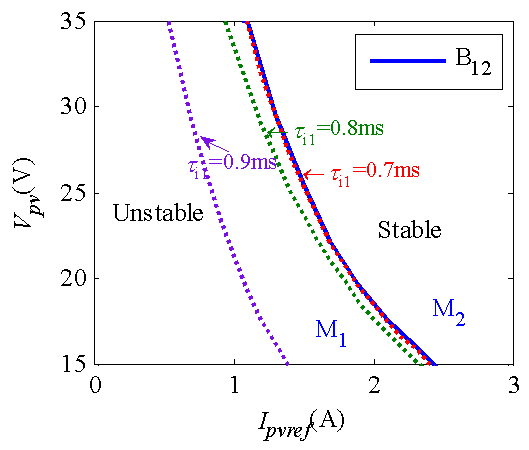(a) Stability boundaries in M1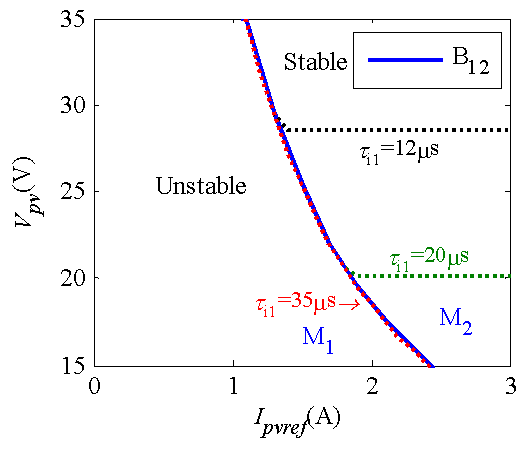(b) Stability boundaries in M2

Figure 5. The stability boundaries of output voltage control loop.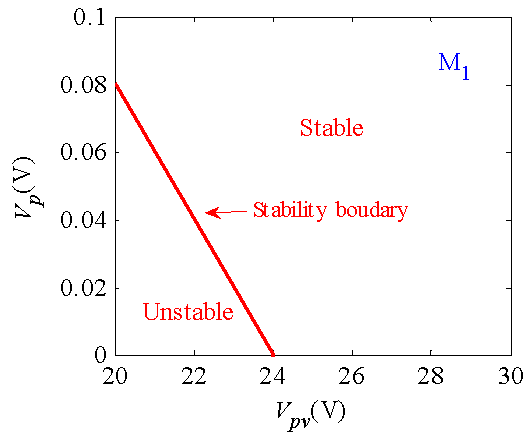(a) The parameter space of Vp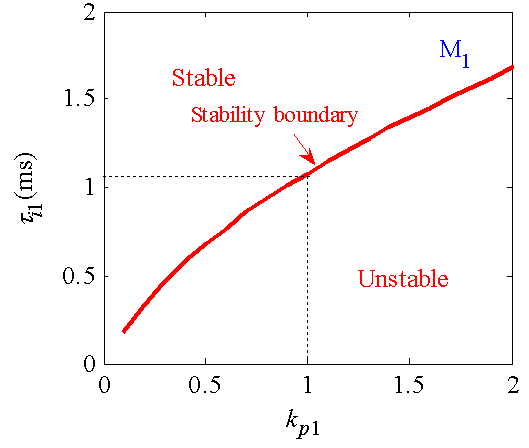(b) The parameter space of "Regulator1"

Figure 6. The parameter space of control parameters at the worst operating condition in M1.

5. Experiment Verification

A 240 W prototype, as shown in Figure 7, was built to verify the nonlinear analysis method based on discrete-time model.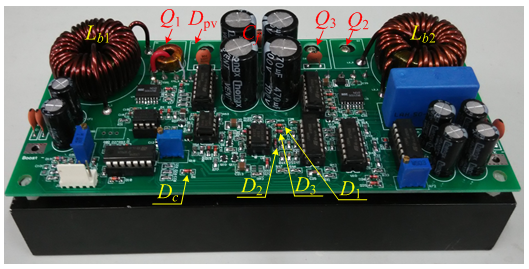Figure 7. Picture of the prototype.

Figure 8(a)-(c) shows the steady-state experiment waveforms at full load in three operating modes when Vpv = 31V. In Figure 8(a) and (b), the waveforms from the top to the bottom are the output voltage Vo; the drive signals of Q1 and Q2, VGS1 and VGS2; inductor current iLb1 and iLb2, respectively. In Figure 8(d), the waveforms are Vo, battery voltage Vbat, iLb1 and iLb2, respectively. From the figure, we can see that the system is stable in three operating modes. When Ipvref= 0.7A, the DC component is ILb1= 5.3A, so Ppv<Po, and the battery provides part of the power to the load, at this time, ILb2> 0. When Ipvref= 3A, suppose the peak value of iLb1 can reach 30A, but the actual peak value is measured to be 14.2A. This is caused by the charge current is controlled and ILb2= −3A, then the PV panels quit MPPT control and operates in constant voltage mode. Under the same condition, if the battery is fully charged, the charge voltage control loop is enabled, so the charge current decreases, as shown in Figure 8(c).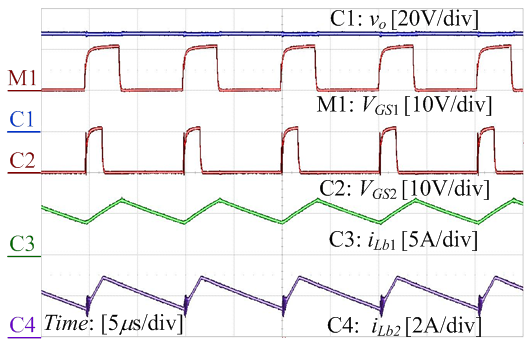(a) M1: Ipvref = 0.7AVocbat = 39V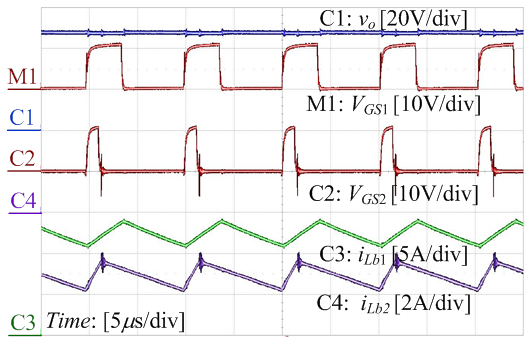(b) M2: Ipvref = 3AVocbat = 39V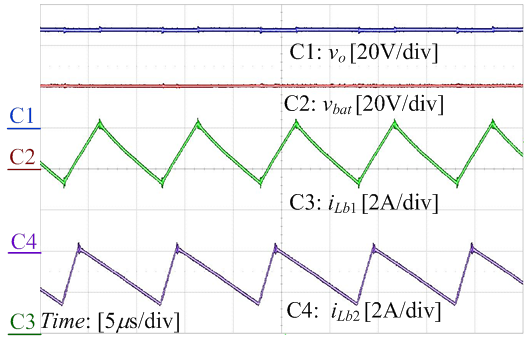(c) M3: Ipvref = 3A, Vocbat = 40V

Figure 8. Steady experiment waveforms in three operating modes.

A step load change is applied to the prototype when Vpv = 30V. Figure 9 shows the experiment waveforms of io, vo, iLb1, iLb2 and vbat under the load stepping up or stepping down between 10% load and full load, where vo and vbat are given as AC component. From Figure 9, we can see that the system can properly respond to the step change and switches its operating mode correctly and keeps stable after the transient process. Take Figure 9(a) as an example, when the load suddenly steps up to full load and then steps down to 10% load, the system switches from M1 to M2 and then switches to M1 again, still keeps stable.

The experiment results show that the control parameters designed with nonlinear analysis method can make the system operate correctly and stably.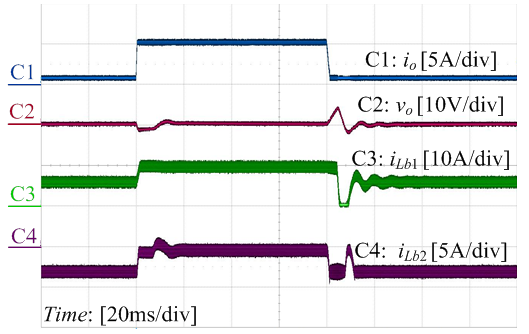(a)      Ipvref = 1.1A (Pm = 280W), Vocbat = 39V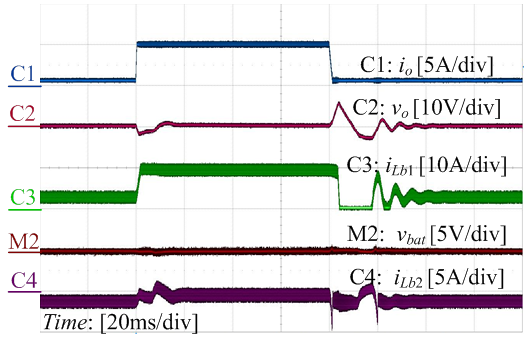(b)      Ipvref = 1.1A (Pm = 280W), Vocbat = 40V

Figure 9. Experiment waveforms of load stepping up and load stepping down.

6. Conclusions

Due to the intermittent nature of the availability of solar power and the bidirectional flow of the battery power, a commonly used standalone photovoltaic-battery hybrid power system is designed to operate with three operating modes. This system is constructed with photovoltaic panels and a battery is connected to the load via a boost converter and a bidirectional buck/boost converter, respectively. As the control loops are coupled with each other in every operating mode and make the closed-loop design become difficult and impossible with small-signal analysis method based on averaged state-space. This paper fixes this problem using the nonlinear analysis method based on discrete-time mapping model, which is performed to find the worst operating condition for each control loop and then evaluate the stability boundaries of the parameters of the closed-loops at the worst operating condition. At last, the parameters of the closed-loop are chosen in the stable region.. Moreover, a prototype is built and the experimental results are shown to verify the nonlinear analysis method in the design of closed-loop.

Acknowledgements

Project is supported by "the Fundamental Research Funds for the Central Universities" (2016BJ0092).

References

1. M. H. Nehrir, C. Wang, K. Strunz, H. Aki, R. Ramakumar, J. Bing, Z. Miao, and Z. Salameh, "A review of hybrid renewable/alternative energy systems for electric power generation: configurations, control, and applications," IEEE Trans. Sustain. Energy,vol. 2, no. 4, pp. 392–403, Oct. 2011.
2. Giraud F, Salameh Z M, "Steady-state performance of a grid-connected rooftop hybrid wind- photovoltaic power system with battery storage," IEEE Trans on Energy Conversion, 2001, 16(1): 1–7.
3. Rahman S, Tam K, "Feasibility study of photovoltaic-fuel cell hybrid energy system," IEEE Trans on Energy Conversion, 1998, 3(1): 50–55.
4. Nehrir M H, Wang C, Strunz, Aki H, Ramakumar R, Bing J, Miao Z, Salameh Z, "A review of hybrid renewable/alternative energy systems for electric power generation: Configurations, control, and applications," IEEE Trans on Sustainable Energy, 2011, 2(4): 392–403.
5. She X,Huang A Q,Lukic S,et al, "On integration of solid-state transformer with zonal DC microgrid," IEEE Trans. on Smart Grid2012,3(2):975-985.
6. KakiganoH,MiuraY,Ise T, "Low-voltage bipolar-type DC microgrid for super high quality distribution," IEEE Trans.on Power Electronics,2010, 25(12): 3066-3075.
7. Zhang J, Ji L, "An effective hybrid energy storage system based on battery-EDLC for distributed generation systems," Proc. of IEEE Conference of Industrial Electronics Application, 2010: 819–824.
8. J. Xiao and P.Wang,"Multiple modes control of household DC microgrid with integration of various renewable energy sources," in IEEE Ind. Electron. Conf. Record, 2013, pp. 1773–1778.
9. C. Zhao, S. D. Round, and J. W. Kolar, "An isolated three-port bidirectional DC-DC converter with decoupled power flow management," IEEE Trans. Power Electron., vol. 23, no. 5, pp. 2443–2453, Sept. 2008.
10. Li Y, Ruan X, Yang D, Liu F, "Modeling, analysis and design for hybrid power systems with dual-input dc-dc converter," Proc. IEEE Energy Conversion Congress and Exposition(ECCE), 2009: 3203–3210.
11. X.Zhang,X.Ruan, andC.K.Tse, "Impedance-based local stability criterion for DC distributed power systems," IEEE Trans. Circ. Syst. I:Reg. Papers, vol. 62, no.3, pp.916925, March2015.
12. C.Wan,M.Huang,C. K. Tse andX. Ruan, "Effects of interaction of power converters coupled via power grid: a design-oriented study," IEEE Trans.Power Electron.,vol.30, no.7, pp.35893600,July2015.
13. X.Sun,Y.Shen,W. Li and H.Wu, "A PWM and PFM hybrid modulated three-port converter for a standalone PV/Battery power system," IEEEJournal of Emerging and Selected Topics in Power Electronics,vol.3, no.4, pp.9841000,December2015.
14. Liu D W, Li H,Marlino L D,"Design of a 6kWmultiple-input bi-directionaldc/dcconverter with decoupled current sharing control for hybrid energy storage elements,"Proc.IEEEApplied Power Electronics Conference and Exposition(APEC),2007:509-513.
15. X. Xiong, C. K. Tse, and X. Ruan, "Bifurcation analysis of standalonephotovoltaic-battery hybrid power system," IEEE Trans. Circ. Syst. I:Reg. Papers, vol. 60, no. 5, pp. 1354–1365, May 2013.
16. C. K. Tse, Complex Behavior of Switching Power Converters.BocaRaton: CRC Press, 2003.
17. T. Esram and P. L Chapman, "Comparison of photovoltaic array maximumpower point tracking techniques," IEEE Trans. Energy Conv., vol.22, no. 2, pp. 439–449, June, 2007.

 Contents 1. 2. 2.1. 2.2. 3. 4. 5. 6.
Article ToolsAbstractPDF(387K)